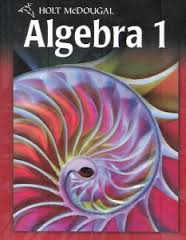# 8-2 Characteristics Of Quadratic Functions

12 Questions | Attempts: 400SettingsOne cannot embark to solve equations without knowing the characteristics. Having covered the characteristics of quadratic equations you are now ready to start working out the problems. The quiz below is from Problems 4-32 even, 41, 42 on pp. 535-537. Take it up and test your knowledge of these characteristics. Good luck!

• 1.
#4, p. 535
• A.

-3

• B.

3

• C.

-3, 3

• D.

No zeros

• 2.
#6, p. 535
• A.
• B.
• C.
• D.

Not here

• 3.
#8, p. 535
• A.
• B.
• C.
• D.

Not here

• 4.
#10, p. 535
• A.
• B.
• C.
• D.

Not here

• 5.
#14, p. 536
• A.
• B.
• C.
• D.

Not here

• 6.
#20, p. 536
• A.

-2

• B.

-1, 1

• C.

2

• D.

Not here

• 7.
#16, p. 536
• A.
• B.
• C.
• D.

Not here

• 8.
#22, p. 536
• A.
• B.
• C.
• D.

Not here

• 9.
#24, p. 536
• A.
• B.
• C.
• D.

Not here

• 10.
#30, p. 536
• A.
• B.
• C.
• D.

Not here

• 11.
#41, p. 537
• A.

A

• B.

B

• C.

C

• D.

D

• 12.
#42, p. 537
• A.

F

• B.

G

• C.

H

• D.

J

## Related TopicsBack to top
×

Wait!
Here's an interesting quiz for you.